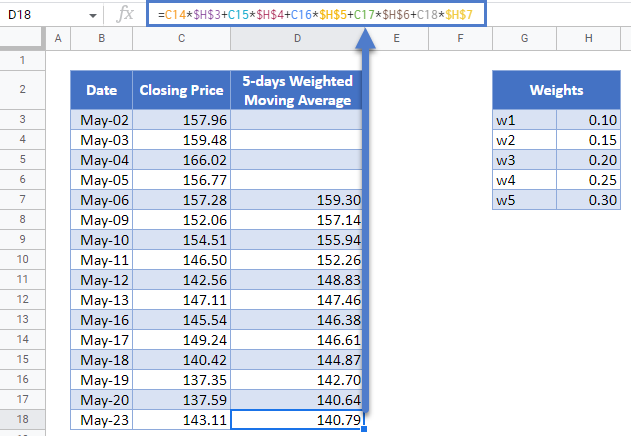# Weighted Moving (Rolling) Average – Excel and Google Sheets

This tutorial will demonstrate how to calculated the weighted moving average in Excel and Google Sheets.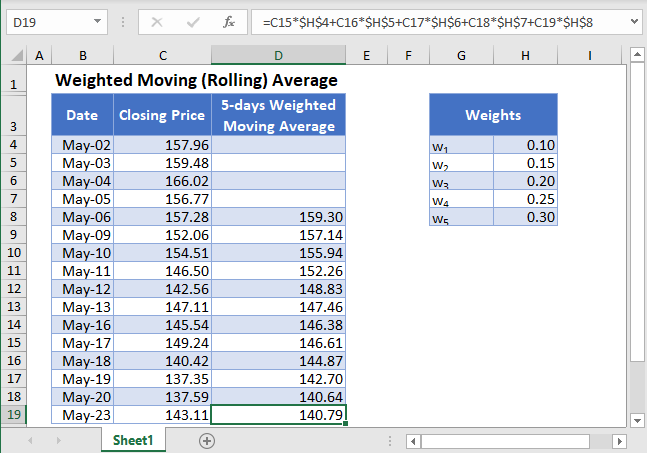Moving (Rolling or Running) Average is a series of averages that shows the averages of different fixed subsets of the entire dataset. For example, a 5-days moving average shows the average of the previous 5-days.Moving Average is used in time series data analysis to smoothen out short-term fluctuations in a dataset and thus be able to highlight and predict longer-term trends or cycles. The moving average can be calculated as a simple, weighted, or exponential moving average.

The Weighted Moving Average calculates the moving average of a subset of  data points where each data point has some weights assigned to them. In this article, we will look at how to calculate the weighted moving average.

## Weighted Moving Average Formula

The -data points weighted moving average (k) is calculated using the formula: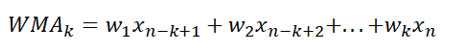where xn-k+1 + xn-k+2 + … + xn are the current data value and the previous k-1  data values;

WMAk is the k-data points weighted moving average of  k data points up to the current data point;

w1, w2,…,wk are the weights of k data points up to the current data points expressed as percentages of the total weight; and

k is the desired fixed subset of the dataset.

## How to Calculate Weighted Moving Average in Excel

Background: The closing prices of Apple Inc. stock on NASDAQ for the sixteen trading days from 2nd to 23rd May 2022 are shown in the table below. Calculate the 5-days moving averages for the stock prices using the weights 0.3, 0.25, 0.2, 0.15, and 0.1 for the current day, the previous day, the next previous day, etc., respectively.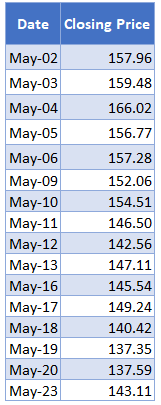First, insert the weights in a different table as shown below.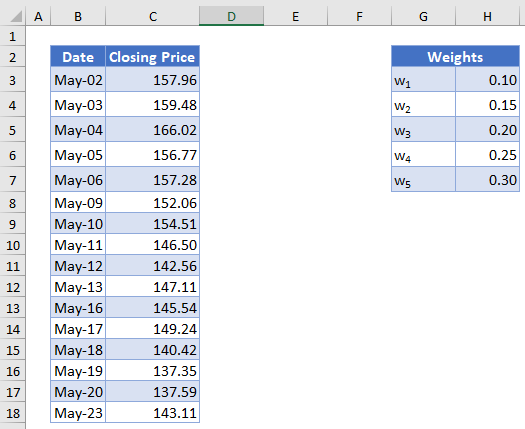Next, calculate the 5-days moving average. We will start from the 5th data, i.e. May-06. Multiply  to the 4th farthest data point,  to the 3rd farthest data point,  to the 2nd farthest data point,  to the previous data point, and  to the current data point. Repeat this process for all the subsequent weighted moving averages. The calculation is shown below.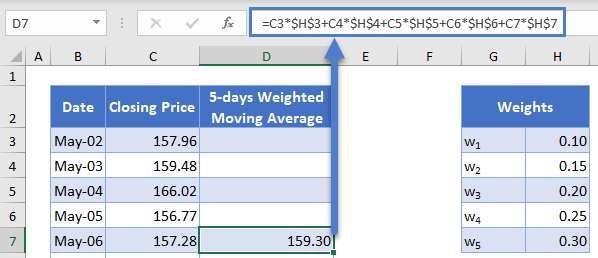Autofill the rest of the column and you have the complete 5-days Weighted Moving Average column as shown below.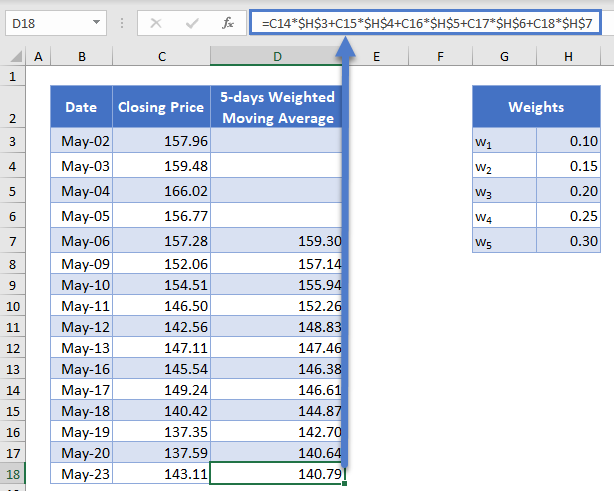## Weighted Moving Average in Google Sheets

Weighted Moving Average can be calculated in Google Sheets in a similar way as it is calculated in Excel as shown in the picture below.